Test volume method

The test volume method provides an alternative to the virial pressure route for calculating the pressure of a system. This method consists of evaluating the change in internal energy,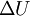$\Delta U$ produced by a small change in the volume of the system,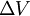$\Delta V$.
This can be thought of as a "blow-up" (or "in") of the whole system,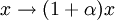$x \rightarrow (1+\alpha) x$ with a small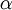$\alpha$ (similarly for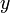$y$ and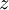$z$), exactly in the same way as the virial pressure expression may be derived, i.e. not as leaving the particles in their places and changing the simulation cell (a procedure which does not make sense in general).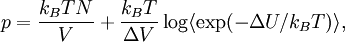$p = \frac{ k_B T N}{V} + \frac{ k_B T }{ \Delta V } \log \langle \exp(-\Delta U/k_B T )\rangle,$
where$k_B$ is the Boltzmann constant and$T$ is the temperature. The method is clearly inspired by the Widom test-particle method, which is used to obtain the chemical potential. It has been argued that this method can be more useful than virial pressure, since it avoids the calculation of forces (which are not needed in a Monte Carlo simulation). Moreover, there are interaction potentials that are discontinuous. In such cases the forces are not well defined, and one would have to consider the limit of "almost-discontinuous" potentials instead, which can be cumbersome. However, it should be noted that it is not clear how to "blow up" a system containing molecules held by rigid constraints.